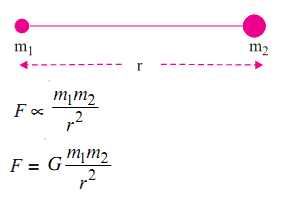# Newton's Law of Gravitation

Newton expressed the law of gravitation in the language of mathematics.

He stated the law as follows:

Every particle in the universe attracts every other particle with a force. This force is proportional to the product of their masses and inversely proportional to the square of the distance between them. The force is along the line joining the two particles.

Mathematically,G is a constant of proportionality. It is called the universal gravitational constant. Its value is same everywhere on the earth or in the universe.

G = 6.67 × 10–11 Nm2kg–2

As the value of G is very small, the force of gravitation between objects of ordinary mass is very weak. However, the force of gravitation becomes appreciably stronger if masses of the objects are increased.

Example 1: A boy of 40 kg mass is standing on the surface of earth. If the mass of the earth is 6 × 1024 kg and its radius is 6.37 ×106 m, then find the force of attraction between the boy and the earth. Take the value of G as 6.67 × 10–11 Nm2 kg–2.

Mass of the earth = 6 × 1024 kg

Mass of the boy = 40 kg

Radius of the earth = 6.37 × 106 m

This is the distance separating the boy from the centre of the earth.

G = 6.67 × 10–11 Nm2kg–2

The force of attraction (F) between the boy and the earth = 394.5 N

The gravitational force due to earth is also known as gravity. Thus, when you are dealing with very large masses like the earth, the moon or the sun, the gravitational force between such objects is quite large.